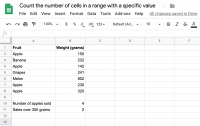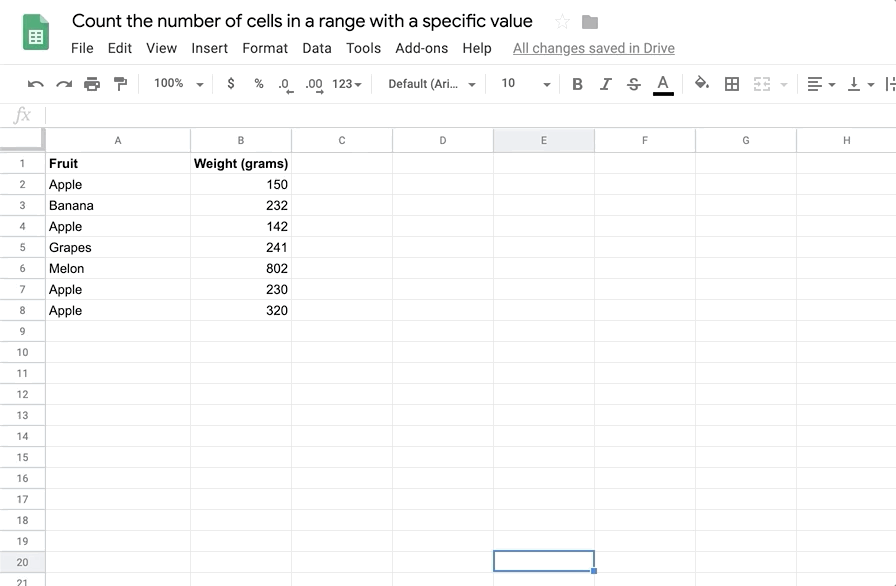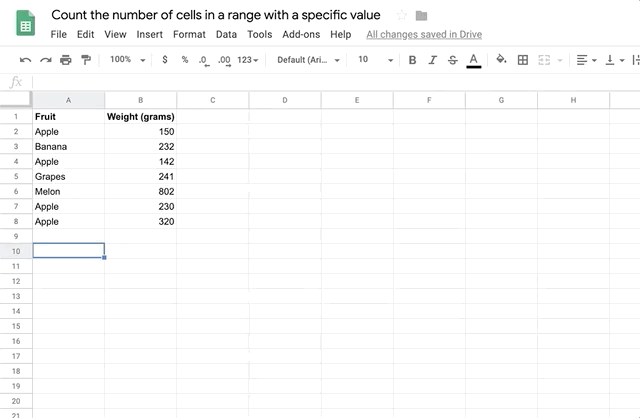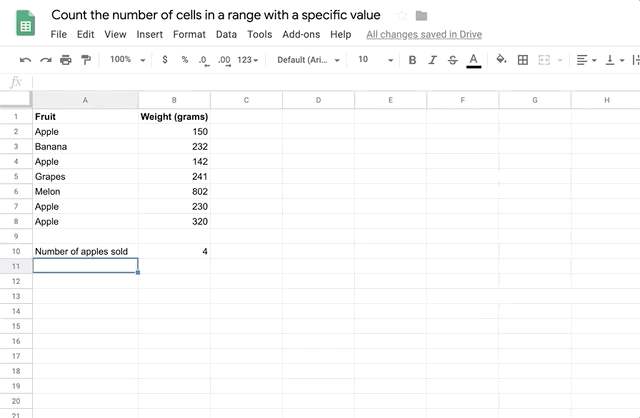# How to count the number of cells in a range with a specific value in Google sheetsTutorial
Dec 11 2019

Sometimes you will want to count the number of cells in a range of values or you might want to count the number of values over a certain amount, or the number of sales of a particular type.

In this tutorial we are going to count the number of cells which contain the word Apple, and the number of cells over a weight of 300 grams. We will do this using the COUNTIF function.

## Function syntax

``=COUNTIF(RANGE, CRITERION)``

## Examples

``=COUNTIF(A1:A10, "=Apple")``
``=COUNTIF(A1:A10, ">100")``Get your sample data ready. You can use the starter data on the first tab of the Google sheets example for this tutorial.

## Count the number of cells using the 'equals' operatorEnter 'Number of apples sold' in A10. Then in B10, enter the following:

``=COUNTIF(A2:A8, "=Apple")``

This formula selects the range (A2:A8) and then counts the number of cells in that range whose value equals 'Apple'

## Count the number of cells using the 'greater-than' operatorNow we will count the number of sales that are over 300 grams in weight. To do this, enter the label 'Sales over 300g' in the cell A11, and enter the formula:

``=COUNTIF(B2:B8, ">300")``

This selects the range B2:B8, and counts the values that are greater than 300.